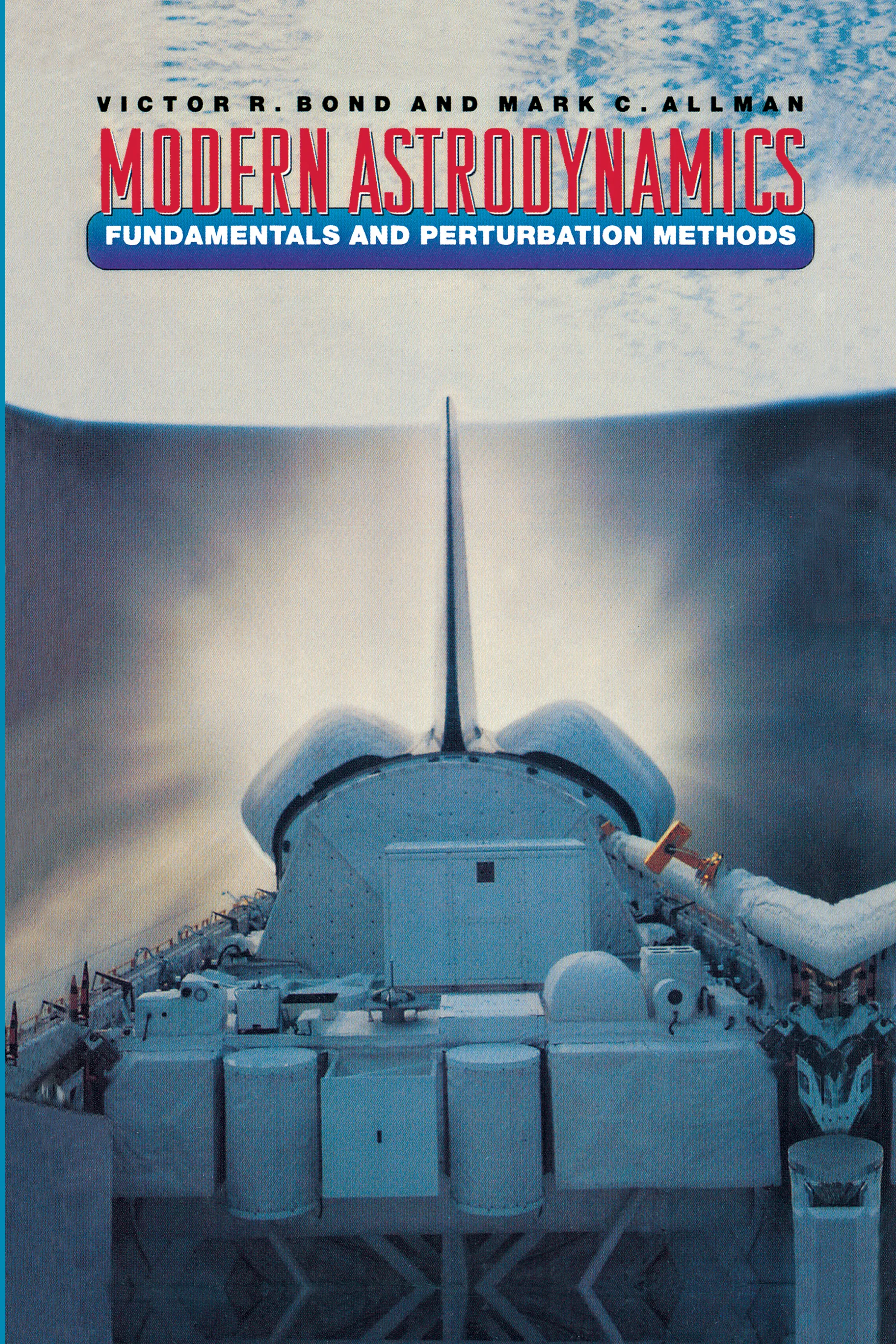#### Modern Astrodynamics

Victor R. Bond

##### ebooks

Many of our ebooks are available for purchase from these online vendors:

Many of our ebooks are available through library electronic resources including these platforms:

# Modern Astrodynamics: Fundamentals and Perturbation Methods## Hardcover

Price:
\$135.00 / £104.00
ISBN:
Published:
Mar 24, 1996
1996
Pages:
264
Size:
6 x 9.25 in.
Illus:
10 tables, 50 halftones

Newton’s laws of motion and his universal law of gravitation described mathematically the motion of two bodies undergoing mutual gravitational attraction. However, it is impossible to solve analytically the equation of motion for three gravitationally interacting bodies. This book discusses some techniques used to obtain numerical solutions of the equations of motion for planets and satellites, which are of fundamental importance to solar-system dynamicists and to those involved in planning the orbits of artificial satellites.

The first part introduces the classical two-body problem and solves it by rigorously developing the six integrals of the motion, starting from Newton’s three laws of motion and his law of gravitation and then using vector algebra to develop the integrals. The various forms of the solution flow naturally from the integrals. In the second part, several modern perturbation techniques are developed and applied to cases of practical importance. For example, the perturbed two-body problem for an oblate planet or for a nonsymmetric rotating planet is considered, as is the effect of drag on a satellite. The two-body problem is regularized, and the nonlinear differential equation is thereby transformed to a linear one by further embedding several of the integrals. Finally, a brief sketch of numerical methods is given, as the perturbation equations must be solved by numerical rather than by analytical methods.